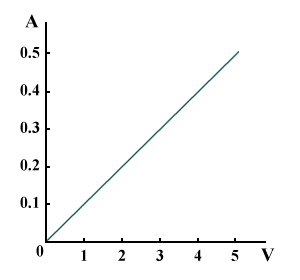# Ohm's Law

The values recorded in the table below show how the current I through a certain conductor varies when there are different voltages V across it. Note that the ratio V/I has a constant value (10), and because V/I = resistance R, it follows that R for the conductor does not change when V and I change. Ohm's law sums up the behaviour of this and similar conductors. It states that the current through a conductor is directly proportional to the voltage (or potential difference) across it, and inversely proportional to its resistance (assuming that the temperature and other physical conditions do not change). Conductors that obey Ohm's law are made of metal, carbon and some alloys. They are called ohmic conductors. Conductors not obeying Ohm's law are non-ohmic - their resistance changes with voltage and current.

How current varies with voltage
Voltage VCurrent IResistance Ω
1 0.1 10
2 0.2 10
3 0.3 10
4 0.4 10
5 0.5 10

A useful graph for a conductor can be obtained by plotting I along the y-axis and V along the x-axis. This is known as a characteristic curve. The characteristic curve for an ohmic conductor is a straight line passing through the origin, 0, of the graph. For this reason, ohmic conductors are also called linear conductors. The axes of the graph are labelled A and V to indicate that current I is measured in amperes and voltage V in volts.A graph of voltage versus current

The following diagram is a useful aid to help you derive an equation for voltage, current, or resistance. To use the VIR triangle place your finger over the value you wish to find. For example, if you wish to find V then multiply I by R. If you wish to find I then divide V by R.Ohm's triangle